##### The Japanese Bonsai specialist
Direct order Contact Help / Services Newsletter# Pinus pentaphylla du japon ref :12090222

› Outdoor bonsai trees › Japanese yamadoris bonsaï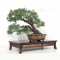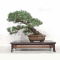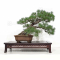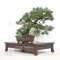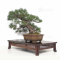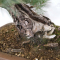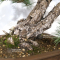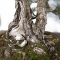ref. : 11773

1.640,00

voluminous/heavy item extra shipping of 12,00

Available quantity : 1Order

###### Description

Height of the only tree : 400 mm.

Width of the branches : 520 mm.

Dimensions of the quality non-enameled firmed pot from Yixing : 300*240*100 mm.

Short and softly blue-toned needles. 2-trunks Sokan style.

There is a shari on the front side of the base of the trunk : beautiful asset of this bonsaï. This bonsaï is more than 50 years old with its formed bark. It comes from yamadori, more than 30 years ago. It is well-ramified and the trunk has a good conicity. No grafting marks since it is a natural seedling, nor strong wire marks or cutting wound.

Diameter of the trunk : 30-45 mm. Superb and regular nebari : 90-130 mm. Quality subject for a collector who will take time to cut it regularly to densify the trays. Admire the natural beauty of its trunk shari. Photographed in September 2022. It comes from Murakawa nursery. The shelf is not included.

#bonsai 4.6 #trunk 4.5 #from 3.4 #pentaphylla 3.1 #yamadoris 2.9 #japanese 2.8 #natural 2.7 #quality 2.7 #pinus 2.5 #marks 2.5

Formule
(( ROUND((CHAR_LENGTH(b.article_nom)-CHAR_LENGTH(REPLACE(b.article_nom, 'trunk', '')))/LENGTH('trunk')) + ROUND((CHAR_LENGTH(b.article_description)-CHAR_LENGTH(REPLACE(b.article_description, 'trunk', '')))/LENGTH('trunk')) ) * 4.5) + (( ROUND((CHAR_LENGTH(b.article_nom)-CHAR_LENGTH(REPLACE(b.article_nom, 'from', '')))/LENGTH('from')) + ROUND((CHAR_LENGTH(b.article_description)-CHAR_LENGTH(REPLACE(b.article_description, 'from', '')))/LENGTH('from')) ) * 3.4) + (( ROUND((CHAR_LENGTH(b.article_nom)-CHAR_LENGTH(REPLACE(b.article_nom, 'pentaphylla', '')))/LENGTH('pentaphylla')) + ROUND((CHAR_LENGTH(b.article_description)-CHAR_LENGTH(REPLACE(b.article_description, 'pentaphylla', '')))/LENGTH('pentaphylla')) ) * 3.1) + (( ROUND((CHAR_LENGTH(b.article_nom)-CHAR_LENGTH(REPLACE(b.article_nom, 'natural', '')))/LENGTH('natural')) + ROUND((CHAR_LENGTH(b.article_description)-CHAR_LENGTH(REPLACE(b.article_description, 'natural', '')))/LENGTH('natural')) ) * 2.7) + (( ROUND((CHAR_LENGTH(b.article_nom)-CHAR_LENGTH(REPLACE(b.article_nom, 'quality', '')))/LENGTH('quality')) + ROUND((CHAR_LENGTH(b.article_description)-CHAR_LENGTH(REPLACE(b.article_description, 'quality', '')))/LENGTH('quality')) ) * 2.7) + (( ROUND((CHAR_LENGTH(b.article_nom)-CHAR_LENGTH(REPLACE(b.article_nom, 'bonsai', '')))/LENGTH('bonsai')) + ROUND((CHAR_LENGTH(b.article_description)-CHAR_LENGTH(REPLACE(b.article_description, 'bonsai', '')))/LENGTH('bonsai')) ) * 2.6) + (( ROUND((CHAR_LENGTH(b.article_nom)-CHAR_LENGTH(REPLACE(b.article_nom, 'pinus', '')))/LENGTH('pinus')) + ROUND((CHAR_LENGTH(b.article_description)-CHAR_LENGTH(REPLACE(b.article_description, 'pinus', '')))/LENGTH('pinus')) ) * 2.5) + (( ROUND((CHAR_LENGTH(b.article_nom)-CHAR_LENGTH(REPLACE(b.article_nom, 'japon', '')))/LENGTH('japon')) + ROUND((CHAR_LENGTH(b.article_description)-CHAR_LENGTH(REPLACE(b.article_description, 'japon', '')))/LENGTH('japon')) ) * 2.5) + (( ROUND((CHAR_LENGTH(b.article_nom)-CHAR_LENGTH(REPLACE(b.article_nom, 'marks', '')))/LENGTH('marks')) + ROUND((CHAR_LENGTH(b.article_description)-CHAR_LENGTH(REPLACE(b.article_description, 'marks', '')))/LENGTH('marks')) ) * 2.5) + (( ROUND((CHAR_LENGTH(b.article_nom)-CHAR_LENGTH(REPLACE(b.article_nom, 'shari', '')))/LENGTH('shari')) + ROUND((CHAR_LENGTH(b.article_description)-CHAR_LENGTH(REPLACE(b.article_description, 'shari', '')))/LENGTH('shari')) ) * 2.5)

## Secure payment## Delivery

Our logistic partners :04 74 55 23 48
Pépinière MAILLOT-BONSAÏ
Le Bois Frazy
01990 RELEVANT - FRANCE
on appointment